##### Actions

(diff) ← Older revision | Latest revision (diff) | Newer revision → (diff)

A linear topology of a ringin which the fundamental system of neighbourhoods of zero consists of the powers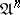of some two-sided ideal. The topology is then said to be-adic, and the idealis said to be the defining ideal of the topology. The closure of any set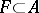in the-adic topology is equal to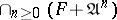; in particular, the topology is separable if, and only if,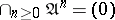. The separable completionof the ringin an-adic topology is isomorphic to the projective limit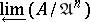.

The-adic topology of an-moduleis defined in a similar manner: its fundamental system of neighbourhoods of zero is given by the submodules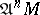; in the-adic topologybecomes a topological-module.

Letbe a commutative ring with identity with an-adic topology and let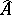be its completion; ifis an ideal of finite type, the topology inis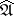-adic, and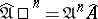. Ifis a maximal ideal, thenis a local ring with maximal ideal. A local ring topology is an adic topology defined by its maximal ideal (an-adic topology).

A fundamental tool in the study of adic topologies of rings is the Artin–Rees lemma: Letbe a commutative Noetherian ring, letbe an ideal in, let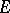be an-module of finite type, and let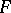be a submodule of. Then there exists asuch that, for any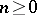, the following equality is valid: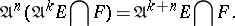The topological interpretation of the Artin–Rees lemma shows that the-adic topology ofis induced by the-adic topology of. It follows that the completionof a ringin the-adic topology is a flat-module (cf. Flat module), that the completion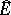of the-moduleof finite type is identical with, and that Krull's theorem holds: The-adic topology of a Noetherian ring is separable if and only if the set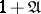contains no zero divisors. In particular, the topology is separable ifis contained in the (Jacobson) radical of the ring.

How to Cite This Entry: